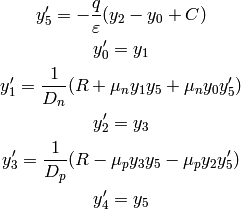# Maxwell’s Equations¶

The Maxwell’s equations are: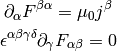and the Lorentz force is: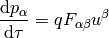where: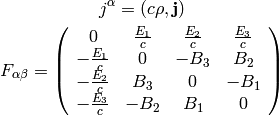This corresponds to: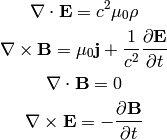## Four Potential¶

The four potential is defined by: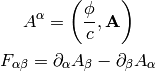this corresponds to: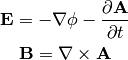The Maxwell’s equations can then be written as (note that the two eq. without sources are automatically satisfied by the four potential):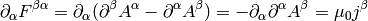where we have employed the Lorentz gauge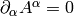.

# Semiconductor Device Physics¶

In general, the task is to find the five quantities: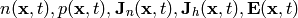where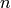(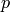) is the electron (hole) concentration,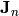(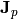) is the electron (hole) current density,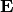is the electric field.

And we have five equations that relate them. We start with the continuity equation: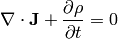where the current density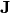is composed of electron and hole current densities: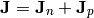and the charge density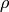is composed of mobile (electrons and holes) and fixed charges (ionized donors and acceptors):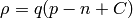whereandis the electron and hole concetration,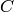is the net doping concetration (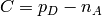where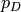is the concentration of ionized donors, charged positive, and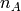is the concentration of ionized acceptors, charged negative) and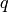is the electron charge (positive). We get: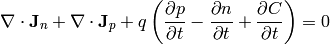Assuming the fixed chargesare time invariant, we get: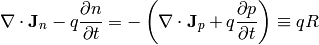where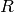is the net recombination rate for electrons and holes (a positive value means recombination, a negative value generation of carriers). We get the carrier continuity equations:

(1)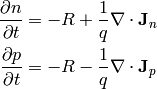Then we need material relations that express how the currentis generated usingandand. A drift-diffusion model is to assume a drift current (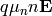) and a diffusion (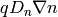), which gives:

(2)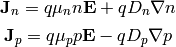where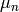,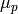,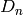,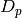are the carrier mobilities and diffusivities.

Final equation is the Gauss’s law: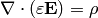(3)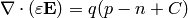## Equations¶

Combining (2) and (1) we get the following three equations for three unknowns,and: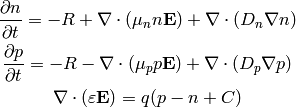And it is usually assumed that the magnetic field is time independent, so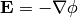and we get:

(4)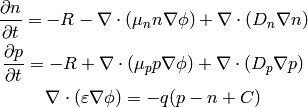These are three nonlinear (due to the terms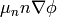and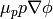) equations for three unknown functions,and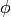.

### Example 1¶

We can substract the first two equations and we get: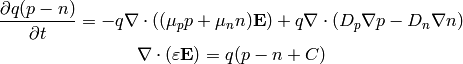and using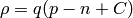and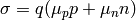, we get: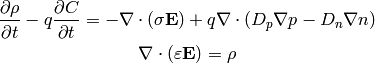So far we didn’t make any assumptions. Most of the times the net doping concetrationis time independent, which gives: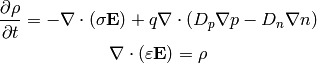Assuming further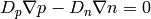, we just get the equation of continuity and the Gauss law: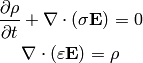Finally, assuming also that thatdoesn’t depend on time, we get: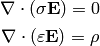### Example 2¶

As a simple model, assume,,,and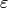are position independent and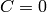,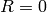: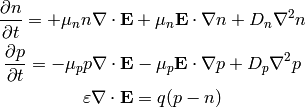Usingwe get: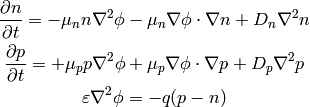## Example 3¶

Let’s calculate the 1D pn-junction. We take the equations (4) and write them in 1D for the stationary state ():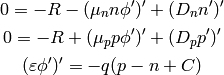We expand the derivatives and assume that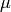and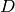is constant: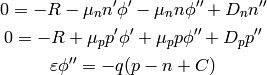and we put the second derivatives on the left hand side:

(5)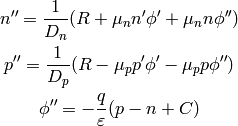now we introduce the variables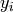: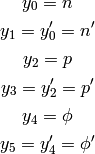and rewrite (5):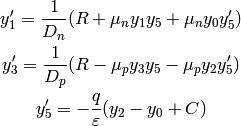So we are solving the following six nonlinear first order ODE:

(6)